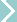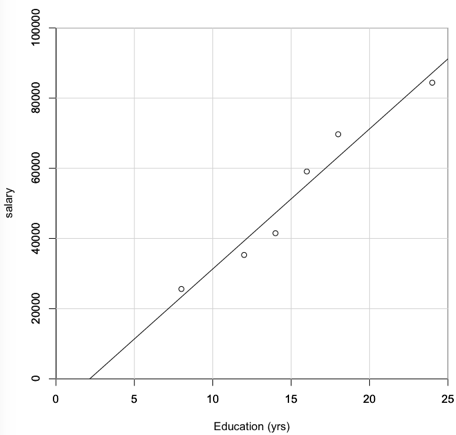Don's HomeProgramming LanguagesR
 Under ConstructionR is a language and environment for statistical computing and graphics. It is a GNU project which is similar to the S language and environment which was developed at Bell Laboratories R is the 6th most common programming language according to IEEE. R provides a wide variety of statistical (linear and nonlinear modelling, classical statistical tests, time-series analysis, classification, clustering, ...). CRAN - Comprehensive R Archive Network - Has packages to extend R. Rstudio is an integrated development environment (IDE) for R. It includes a console, syntax-highlighting editor, as well as tools for plotting, history, debugging and workspace management. I used S briefly while at the Labs and unfortunately R still has the UNIXy obtuse command structure that gives it a steep learning curve. Example: ```Read a csv (Comma separated values) file, plot and compute correlation. sal-edu.csv contains Edu,Sal 8,25600 12,35300 14,41500 16,59100 18,69700 24,84400 ``` ```> test <- read.csv(file="/Users/donmcbride/Programs/sal-edu.csv",head=TRUE,sep=",") > education <- test\$Edu > salary <- test\$Sal > opt <- options("scipen" = 10) # Don't use scientific notation * > plot (education, salary, xlim=c(0, 25), ylim=c(0, 100000), xlab="Education (yrs)", yaxs="i", xaxs="i") > # xaxs="i" causes x 0 point to line up with y axis > axis(1, tck=1, col.ticks="light gray") #adds grid lines > axis(2, tck=1, col.ticks="light gray") > abline(lm(salary ~ education)) # Plot linear regression line> cor (salary, education) # calculate correlation (1 = perfect correlation, 0 = random)  0.98 > q() # quit ``` Some quirks: * scipen = 10 - Use fixed notation "60,000" unless it is more than 10 digits then use scientific notation "6 e4". It defaults to 4 or 5. opt <- options("scipen" = 10) By default ranges are enlarged by 6%, so that the specified values do not lie at the very edges of the plot region. To get 0 to line up with the axis, you need to set the style of the axis interval calculation with xaxs and yaxs. xaxs="i" sets it to "interval" so 0 lines up. See Set R plots x axis to show at y=0 - Stack Overflow When I put a "\$" on the salary amounts it messed up the plot. Apparently it thinks it's an alphanumeric field. I haven't figured out how to fix this. For those from a C background, R provides a wrapper for the sprintf C-library function. e.g. sprintf("\$%.2f", 99.999) Help sights: Quick-R - Help to overcome the steep learning curve for R. Rnoweb: Literate Programming with and for R - Ross Ihaka R-bloggers | R news and tutorials contributed by (580) R bloggers r faq - How to get help in R? - Stack Overflow Enhancements-Libraries: CRAN - Comprehensive R Archive Network - Has packages to extend R. Rstudio is an integrated development environment (IDE) for R Other: Earnings and unemployment rates by educational attainment | bls.gov used for sample data. About Statistics. last updated 11 Oct 2016